Chapter 8

As we know, the magnetic field energy density is: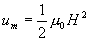(8.1)

Integrating energy density to volume of the sphere of radius r, from the magnetic field equation (5.2), then we can get the electron magnetic field energy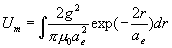(8.2)

Thus: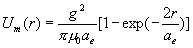(8.3)

The equation (8.3) is the magnetic field energy within the sphere of the radius r.

From equation (8.3), We find out that the magnetic field energy of electron has the spherical exponential distribution.

When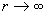, thus: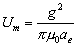(8.4)

Equation (8.4) is the electron magnetic field energy in total.